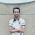### Sieve of Eratosthenes

Sieve of Eratosthenes

(by Anurag Phadnis)

Greetings everyone in this tutorial I will write about sieve of Eratosthenes. It is a very useful algorithm to find all primes which are less than N.

Suppose you want to find all the primes between 1 and 100 how would you do it?
A simple way is to just iterate over all integers between 1 and 100 and check if each integer is prime and print it, if it is prime.
Go to code
But as you can see this algorithm takes too long to run if you need to check if x is prime then you need √(x) operations, and to do that for N times you need N*( Σ√i) {i ε integers between 1 and N}.

The question is "can we do better?"

And yes we can find all the primes between 1 and N in lower time complexity. Let's see how to do that.

Suppose you need to find all prime numbers less than 20, then build a Boolean array that will store 1 if i is prime and 0 if i is not prime.(A[i]=1 if i is prime)

Mark 1 as not prime because one is not prime.
Now a prime number is that number which can be divided only by 1 and itself. Let's look it the other way if a number is divisible by any number other than 1 and itself then that number is not a prime.Now we will mark all the multiples of any number less than N as not prime.

So let's mark all the multiples of 2 as not prime first
The array will now become
1 2 3 4 5 6 7 8 9 10 11 12 13 14 15 16 17 18 19 20
Now mark all multiples of 3 as not prime
1 2 3 4 5 6 7 8 9 10 11 12 13 14 15 16 17 18 19 20
Then mark all multiples of 4 as not prime
1 2 3 4 5 6 7 8 9 10 11 12 13 14 15 16 17 18 19 20

Now as you can see all non primes are marked as red and the numbers left unmarked are primes.
Why marked till 4? For numbers > √N Its multiples will be already marked

This is implementation in c++
Go to code
We take an array of size N+1, here N=100
Now first we don't know which number is prime so we set every number as prime.
Now for all integers less than √N we find all the multiples of that integer which are less than N and mark them as not prime.
Finally we get an array in which all those numbers which are prime are marked as 1 and non primes are marked as zero.

### Comments

1.This comment has been removed by the author.

2.Hey! Nice Code.
But do we need to make this algorithm so complex no one can understand.
I don't think you need to use array at all.
Check my code it is not limited to 100 only and appreciate your work!

#include
int main() {
int a,b;
printf("Enter any Number: ");
scanf("%d",&a);
if(a==1||a==3){
printf("Number is Prime");
}
else{
b=a-3;
if(b%2==0){
printf("Number is Prime");
}
else{
printf("Number is even");
}
}
return 0;
}

1.Appreciate your effort to read the blog and write and comment. Check your code for a=9,a=1,a=2.
Your code will print prime for all the odd numbers, and even for all even numbers,and for a=2 it will have negative mod.

2. Sieve of Eratosthenes is not limited to 100 in that example you can replace it with N take input of N from user and and it will then work till N.

And for that "no one can understand part" please read it once again and let me know which part(or everything) that you didn't understood:)

3.Along with replacing 100 with N also replace 11 with √N.

4.This comment has been removed by the author.